# Calculate Cumulative Totals with Excel SUM Function

Today, through a simple example, we will show you how to use one of the most common-used Mathematical functions in excel, the SUM function, to add up the sum.

In our daily life, we keep an account of what we spend every day. For statistical purpose, we would like to know the total expenses in a period containing several consecutive days. The total expense is the expense of the day plus the expense of the previous days. As time passes, this value is accumulated. Since the total expense is dynamic and accumulated day by day, so to calculate the total spending of a period, we can use Excel SUM function with a mix reference.

Look at the example below. To calculate the total expense for a week, the starting day is Monday, and the ending day is Sunday. The user can check the total expense for any “consecutive days from Monday” of the week in the “Total” column.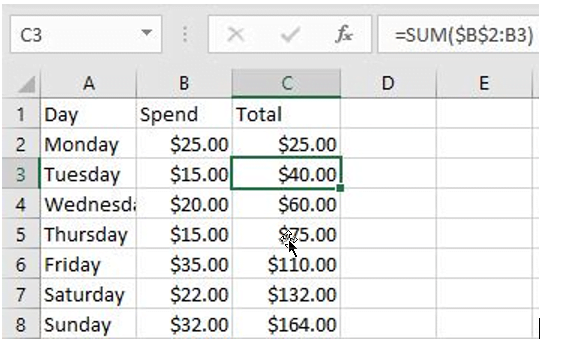In this example, the value in the “Total” column is the total expenses from Monday to that day.

## FORMULA

To sum a range with a locked starting cell, we can use Excel SUM function with a mixed reference. In this example, Monday through Sunday expenses are listed in the “Spend” column, the reference range is B2: B8. The starting day of a week is Monday, so the starting cell for calculating total expense is B2.

In this example, the formula is:

`=SUM(\$B\$2:B2)`

## EXPLANATION

In cell C2, the formula is =SUM(\$B\$2:B2).

Range \$B\$2:B2 only contains cell B2, so the value in B2 is returned in C2 directly.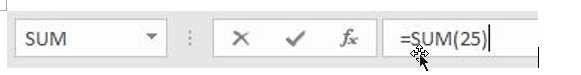Copy the formula down to C3 and the formula is updated to =SUM(\$B\$2:B3).

You can notice that after copying the formula by dragging the handle down, the starting cell is still B2. We lock this cell by adding \$ in front of the row and column indexes. Therefore, if the formula is copied down, this starting cell will not be changed.

The mixed reference is now correctly extended to \$B\$2:B3. So, the values in B2 and B3 are added up.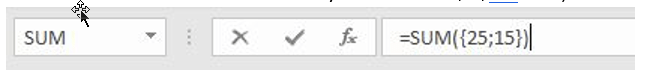As the formula was copied down, B2 was expanded to B3, B4 and up to B8 finally. the spending range was expanded to B8 at the end of the week.

• To calculate the total expense of Monday, Tuesday and Wednesday, the formula is =SUM(\$B\$2:B4).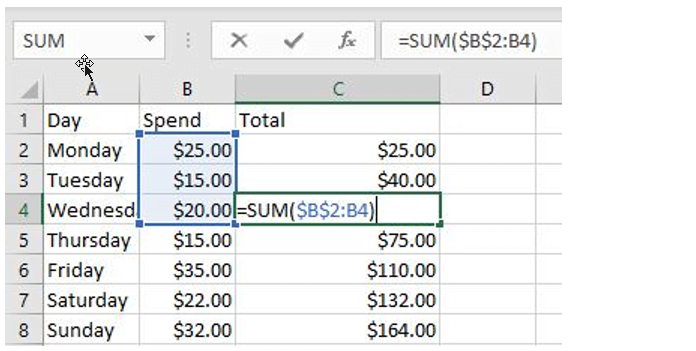• To calculate the total expense of a whole week, the formula is =SUM(\$B\$2:B8).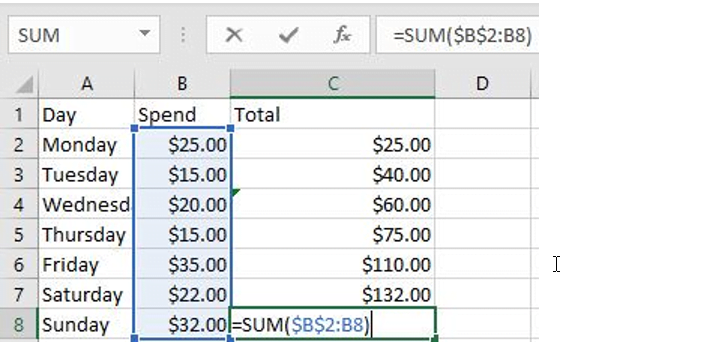### Related Functions

• Excel SUM function
The Excel SUM function will adds all numbers in a range of cells and returns the sum of these values. You can add individual values, cell references or ranges in excel.The syntax of the SUM function is as below:= SUM(number1,[number2],…)…